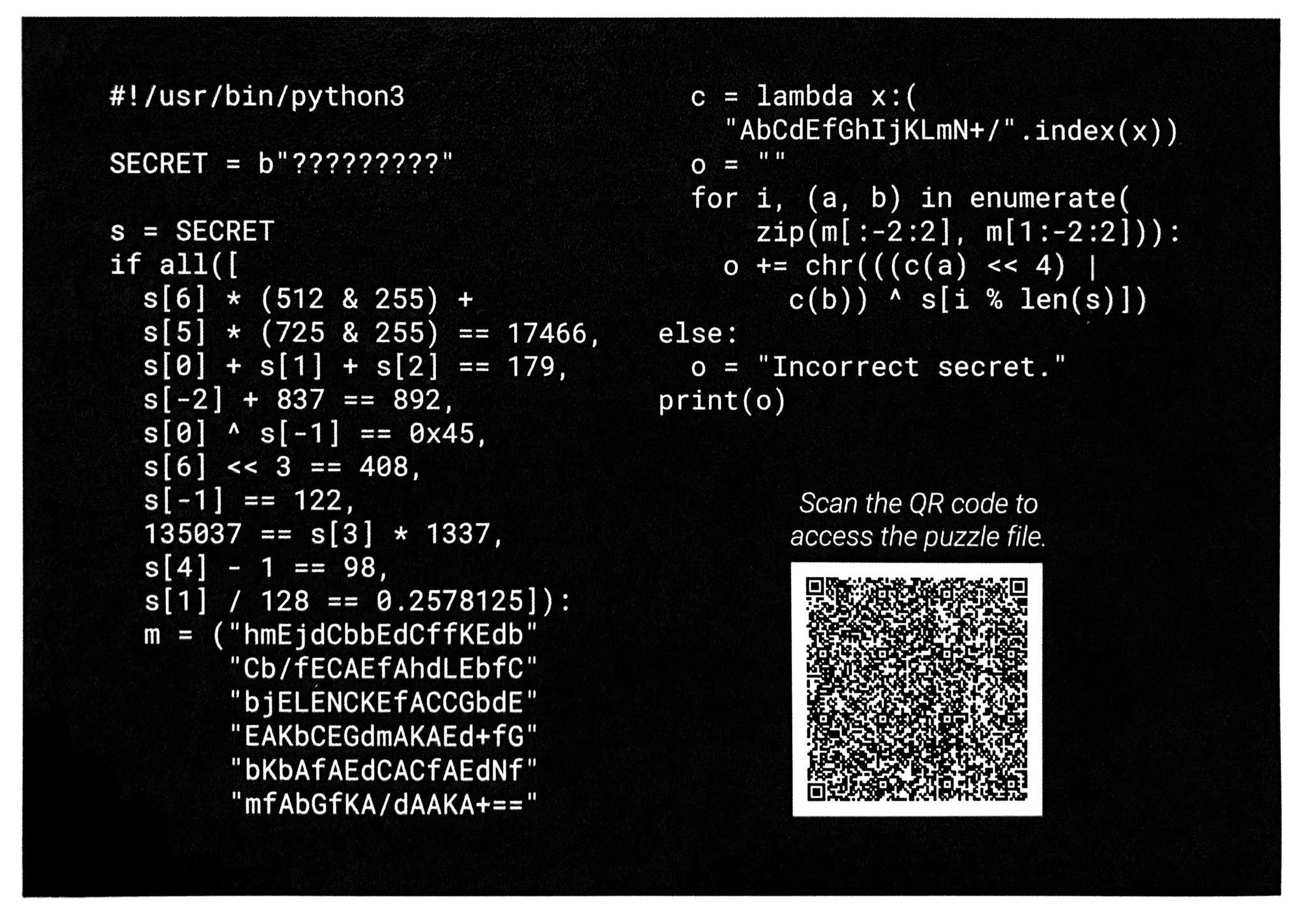# A Challenge from BSidesSF2020

## Google Security & Privacy Engineering Challenge

I stopped by the Google booth at BSidesSF 2020 this weekend, and I picked up a challenge card and a free Titan Security Key Bundle (which I greatly appreciated). Today, I sorted through all the papers, stickers, and t-shirts that I recieved at BSides and RSA, and I rediscovered this card today and solved the challenge. I did censor my answer here though ;)``````#!/usr/bin/python3

#	   012345678
SECRET = b"?????????"

s = SECRET
if all([
s * (512 & 255) +
s * (725 & 255) == 17466,	# s * 213 == 17466, s == chr(82) == 'R'
s + s + s == 179,	# 63 + 33 + s = 179, s == 83, chr(83) == 'S'
s[-2] + 837 == 892,		# s == 892-837 == 55 == '7'
s ^ s[-1] == 0x45,		# s ^ 122 == 0x45, 0x45 == 69, s = 69 ^ 122 == 63, chr(63)=='?'
s << 3 == 408,		# 408 >> 3 == 51, s = chr(51) == '3'
s[-1] == 122,			# chr(122) == 'z', s == 'z'
135037 == s * 1337,		# 135037/1337 == 101, s == chr(101) == 'e'
s - 1 == 98,			# s == chr(99) == 'c'
s / 128 == 0.2578125		# 0.2578125*128 = 33, chr(33) == '!', s == !
]):
m = ("hmEjdCbbEdCffKEdb"
"Cb/fECAEfAhdLEbfC"
"bjELENCKEfACCGbdE"
"EAKbCEGdmAKAEd+fG"
"bKbAfAEdCACfAEdNf"
"mfAbGfKA/dAAKA+==")
c = lambda x:("AbCdEfGhIjKLmN+/".index(x))
o = ""
for i, (a, b) in enumerate(zip(m[:-2:2], m[1:-2:2])):
o += chr( ( (c(a) << 4) | c(b) ) ^ s[i % len(s)] )
else:
o = "Incorrect secret."
print(o)

``````